JAJSGG2C October   2018  – June 2019

PRODUCTION DATA.

1. 特長
2. アプリケーション
3. 概要
4. 改訂履歴
5. 概要（続き）
6. Device Comparison Table
7. Pin Configuration and Functions
8. Specifications
9. Parameter Measurement Information
10. 10Detailed Description
1. 10.1 Overview
2. 10.2 Functional Block Diagram
3. 10.3 Feature Description
1. 10.3.1 Input Range
1. 10.3.2.1 ESD Diodes
2. 10.3.2.2 Input Multiplexer
3. 10.3.3 Programmable Gain Amplifier (PGA)
4. 10.3.4 Reference Voltage
5. 10.3.5 Current Sources (IDAC1 and IDAC2)
6. 10.3.6 General-Purpose Inputs and Outputs (GPIOs)
8. 10.3.8 Digital Filter
4. 10.4 Device Functional Modes
1. 10.4.1 Conversion Control
2. 10.4.2 Auto-Zero Mode
3. 10.4.3 Clock Mode
4. 10.4.4 Reset
5. 10.4.5 Calibration
5. 10.5 Programming
1. 10.5.1 Serial Interface
3. 10.5.3 Conversion Data
4. 10.5.4 Cyclic Redundancy Check (CRC)
5. 10.5.5 Commands
6. 10.6 Register Map
11. 11Application and Implementation
1. 11.1 Application Information
2. 11.2 Typical Applications
2. 11.2.2 Thermocouple Input With High Common-Mode Voltage
3. 11.3 Initialization Setup
12. 12Power Supply Recommendations
13. 13Layout
14. 14デバイスおよびドキュメントのサポート
15. 15メカニカル、パッケージ、および注文情報

• RHB|32
• RHB|32

### 8.8 Typical Characteristics

at TA = 25°C, HV_AVDD = 15 V, HV_AVSS = –15 V, AVDD = 5 V, DVDD = 3.3 V, VREF = 2.5 V, fCLK = 7.3728 MHz, data rate = 20 SPS, and gain = 1 (unless otherwise noted)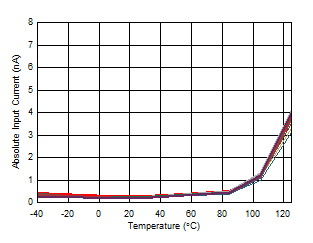V(AINx) = 0 V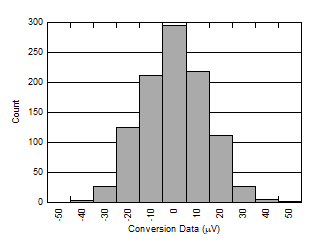Gain = 0.1875, data rate = 1200 SPS, sinc1 filter, calibrated offset, en = 13.6 µVRMS
Figure 8. Noise Histogram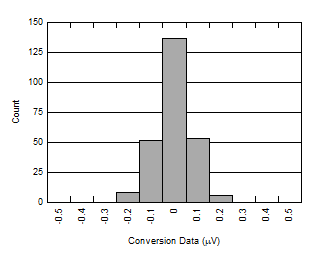Gain = 32, data rate = 20 SPS, FIR filter, calibrated offset, en = 0.076 µVRMS
Figure 10. Noise Histogram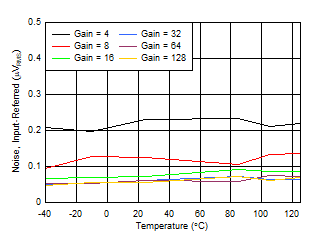Gain = 4 to 128, data rate = 20 SPS
Figure 12. Noise vs Temperature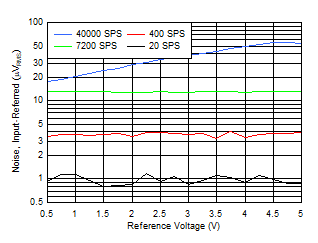Gain = 1
Figure 14. Noise vs Reference VoltageFigure 16. Nonlinearity vs Input Signal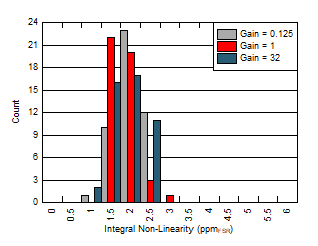Figure 18. Integral Nonlinearity Distribution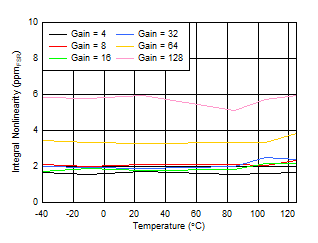Figure 20. Integral Nonlinearity vs TemperatureInput range limited to: ±4 V / Gain when VREF > 4 V
Figure 22. Integral Nonlinearity vs Reference Voltage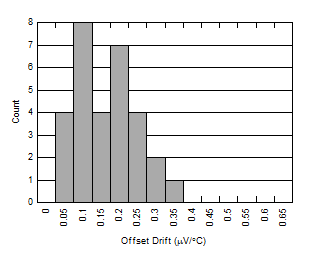Gain = 1
Figure 24. Offset Voltage Drift Distribution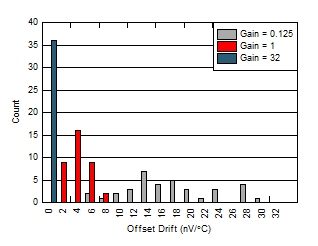Auto-zero mode
Figure 26. Offset Voltage Drift Distribution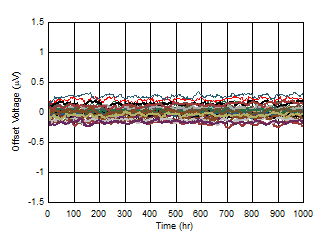32 units, gain = 32, after calibration
Figure 28. Offset Voltage Long-Term Drift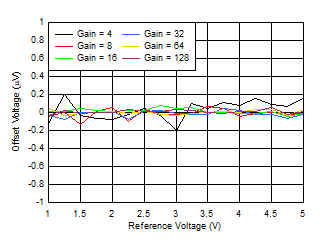Gain = 4 to 128, after calibration
Figure 30. Offset Voltage vs Reference Voltage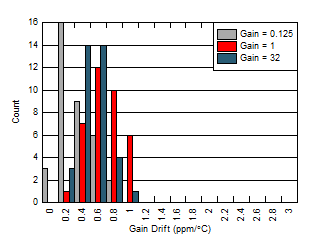Figure 32. Gain Drift Distribution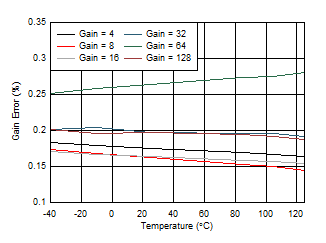Gain = 4 to 128
Figure 34. Gain Error vs Temperature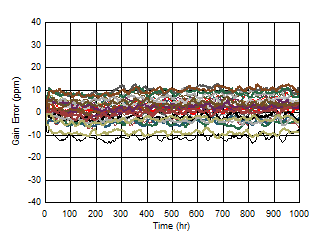32 units, gain = 32, after calibration
Figure 36. Gain Long-Term Drift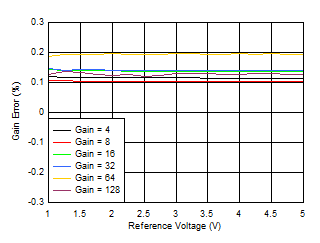Measurement input range limited to: ±4 V / Gain when VREF > 4 V
Figure 38. Gain Error vs Reference Voltage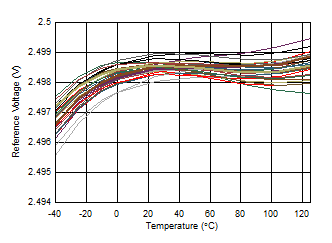Figure 40. Internal Reference Voltage vs Temperature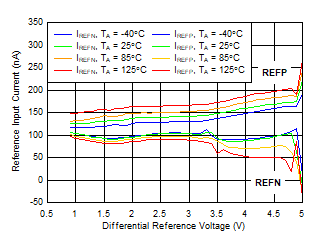Figure 42. Reference Input Current vs Reference Voltage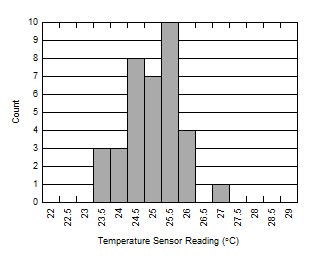TA = 25°C
Figure 44. Temperature Sensor Reading Distribution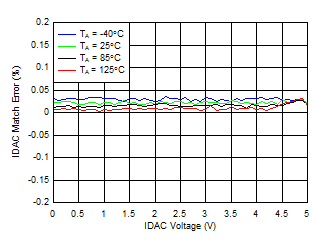IDAC = 250 µA
Figure 46. IDAC Match Error vs IDAC Voltage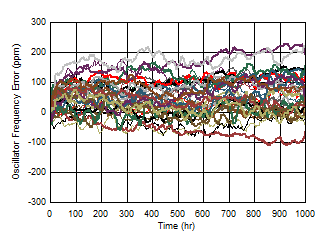32 units, normalized data
Figure 48. Oscillator Frequency Long-Term Drift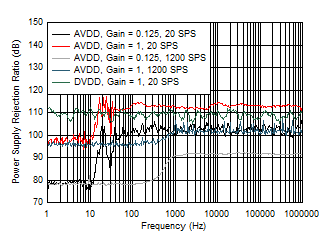AVDD and DVDD
Figure 50. PSRR vs Frequency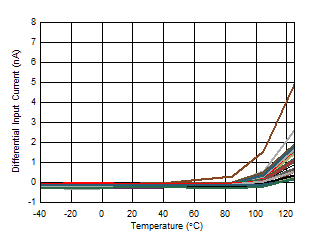VIN = 2.5 V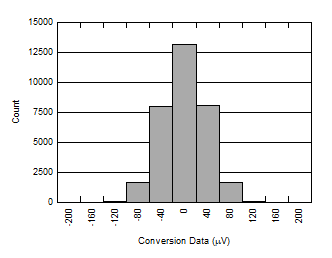Gain = 1, data rate = 40000 SPS, calibrated offset, en = 37 µVRMS
Figure 9. Noise Histogram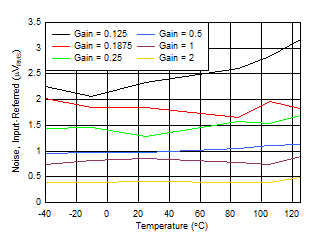Gain = 0.125 to 2, data rate = 20 SPS
Figure 11. Noise vs Temperature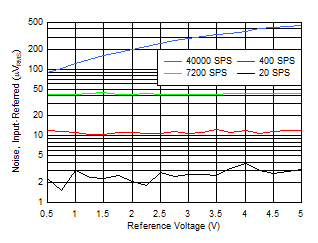Gain = 0.125
Figure 13. Noise vs Reference Voltage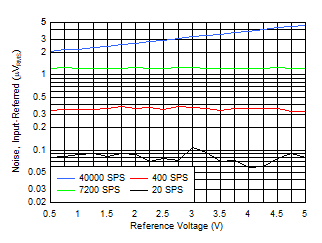Gain = 16
Figure 15. Noise vs Reference Voltage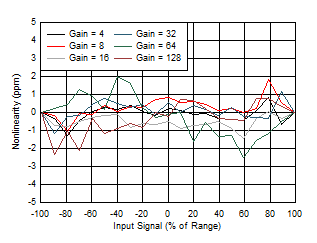Figure 17. Nonlinearity vs Input SignalFigure 19. Integral Nonlinearity vs Temperature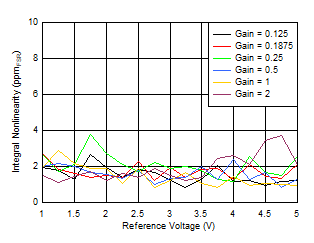Input range limited to: ±4 V / Gain when VREF > 4 V
Figure 21. Integral Nonlinearity vs Reference Voltage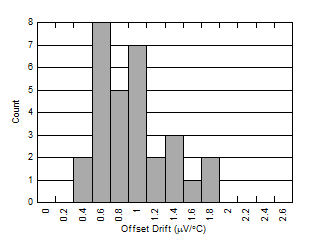Gain = 0.125
Figure 23. Offset Voltage Drift Distribution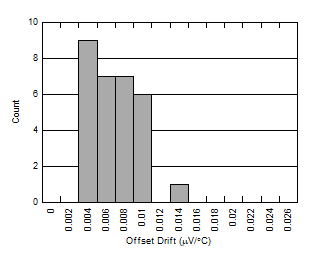Gain = 32
Figure 25. Offset Voltage Drift Distribution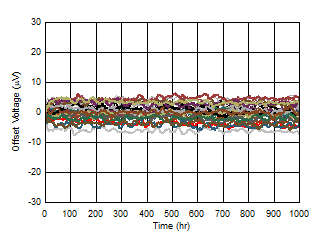32 units, gain = 0.1875, after calibration
Figure 27. Offset Voltage Long-Term Drift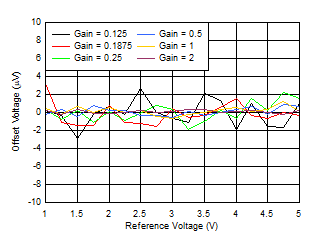Gain = 0.125 to 2, after calibration
Figure 29. Offset Voltage vs Reference Voltage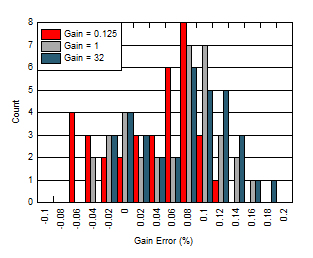Figure 31. Gain Error Distribution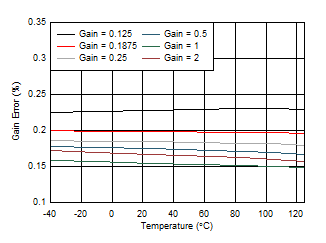Gain = 0.125 to 2
Figure 33. Gain Error vs Temperature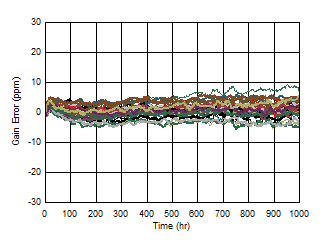32 units, gain = 0.1875, after calibration
Figure 35. Gain Long-Term Drift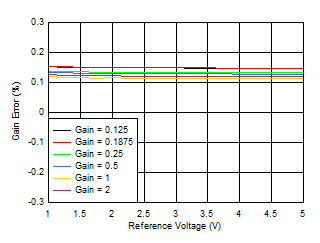Measurement input range limited to: ±4 V / Gain when VREF > 4 V
Figure 37. Gain Error vs Reference Voltage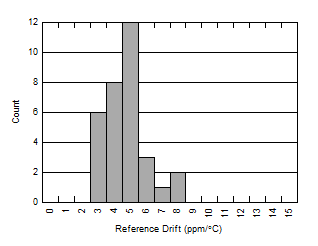TA = –40°C to +125°C
Figure 39. Internal Reference Voltage Drift Distribution32 units, normalized data
Figure 41. Internal Reference Voltage Long-Term Drift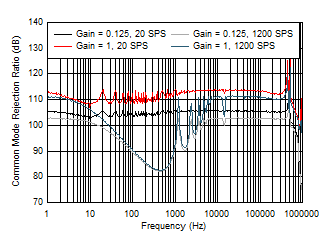Figure 43. Common-Mode Rejection Ratio vs Frequency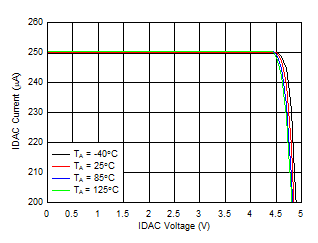IDAC = 250 µA
Figure 45. IDAC Current vs IDAC Voltage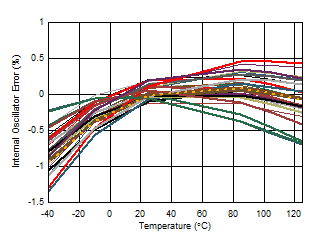Figure 47. Oscillator Frequency Error vs Temperature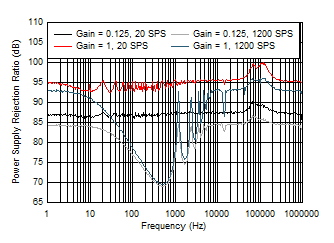HV_AVDD and HV_AVSS
Figure 49. PSRR vs Frequency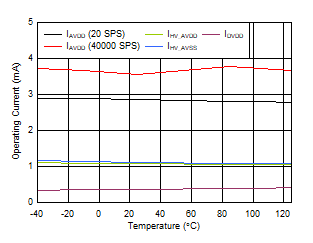All gains
Figure 51. Operating Current vs Temperature